Support Vector Machine (SVM) is an algorithm used for classification problems similar to Logistic Regression (LR). LR and SVM with linear Kernel generally perform comparably in practice. The goal of this article is to compare Support Vector Machine and Logistic Regression.

## Table of Contents

• Introduction
• What is Support Vector Machine?
• What is Logistic Regression?
• Loss functions
• Main Differences
• General Advice
• Take home message
• Conclusion
• References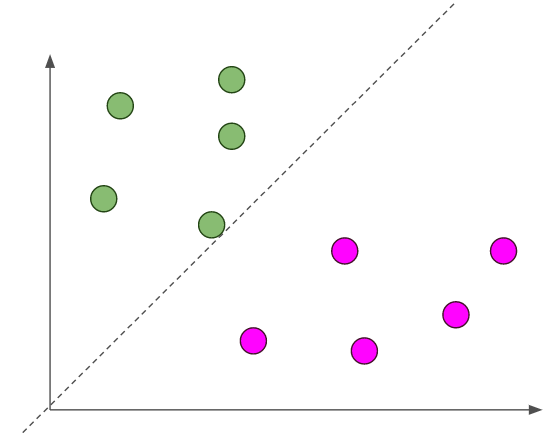-

## Introduction

Given a binary classification problem, the goal is to find the “best” line that has the maximum probability of classifying unseen points correctly. How you define this notion of “best” gives you different models like SVM and logistic regression (LR).

## What is Support Vector Machine?

The objective of the support vector machine algorithm is to find the hyperplane that has the maximum margin in an N-dimensional space(N — the number of features) that distinctly classifies the data points.

Data points falling on either side of the hyperplane can be attributed to different classes. Also, the dimension of the hyperplane depends upon the number of features. If the number of input features is 2, then the hyperplane is just a line. If the number of input features is 3, then the hyperplane becomes a two-dimensional plane.

Support vectors are data points that are closer to the hyperplane and influence the position and orientation of the hyperplane. Using these support vectors, we maximize the margin of the classifier. Deleting the support vectors will change the position of the hyperplane. These are the points that help us build our SVM.

## What is Logistic Regression?

In logistic regression, we take the output of the linear function and squash the value within the range of [0,1] using the sigmoid function( logistic function). The Sigmoid-Function is an S-shaped curve that can take any real-valued number and map it into a value between the range of 0 and 1, but never exactly at those limits. Typically, if the squashed value is greater than a threshold value we assign it a label 1, else we assign it a label 0. This justifies the name ‘logistic regression’.
Note that the difference between logistic and linear regression is that Logistic regression gives you a discrete outcome but linear regression gives a continuous outcome.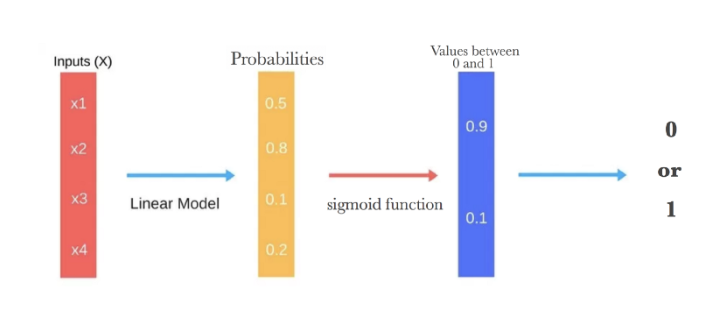Steps that logistic regression goes through to give you your desired output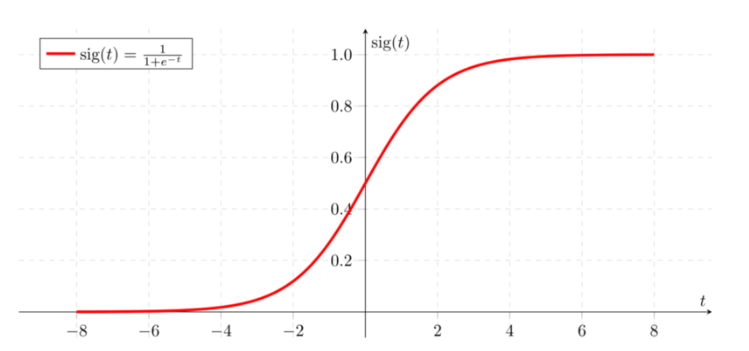Sigmoid Activation Function

We want to maximize the likelihood that a random data point gets classified correctly, which is called Maximum Likelihood Estimation. Maximum Likelihood Estimation is a general approach to estimating parameters in statistical models. You can maximize the likelihood using different methods like an optimization algorithm (Newton’s Method, Gradient Descent etc).

## Loss functions

This definition of “best” results in different loss functions. If you look at the optimization problems of linear SVM and (regularized) LR, they are very similar:

That is, they only differ in the loss function — SVM minimizes hinge loss while logistic regression minimizes logistic loss.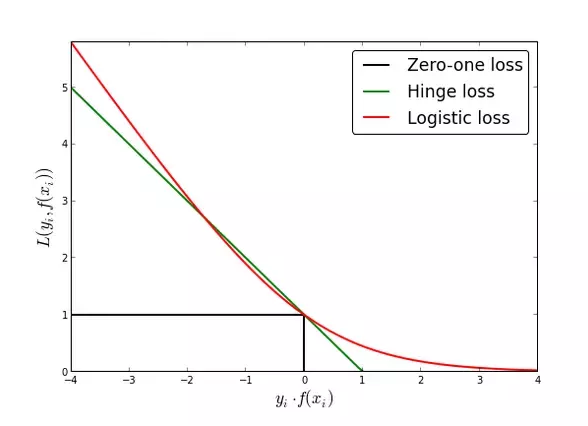Loss functions

There are 2 differences to note:

• Logistic loss diverges faster than hinge loss. So, in general, it will be more sensitive to outliers.
• Logistic loss does not go to zero even if the point is classified sufficiently confidently. This might lead to minor degradation in accuracy.

So, you can typically expect SVM to perform marginally better than logistic regression.

🚀 For people who like video courses and want to kick-start a career in data science today, I highly recommend the below video course from Udacity:

📚 While for book lovers:

## Main Differences

• SVM try to maximize the margin between the closest support vectors while LR the posterior class probability. Thus, SVM find a solution which is as fare as possible for the two categories while LR has not this property.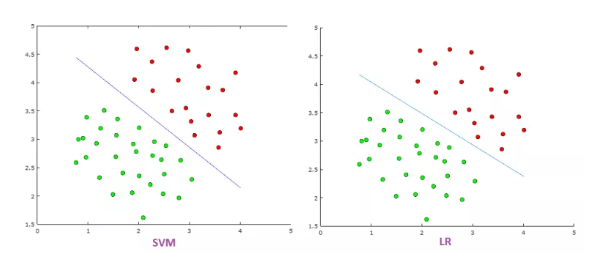SVM has a more balanced boundary between two categories
• LR is more sensitive to outliers than SVM because the cost function of LR diverges faster than those of SVM. So putting an outlier on above picture would give below picture: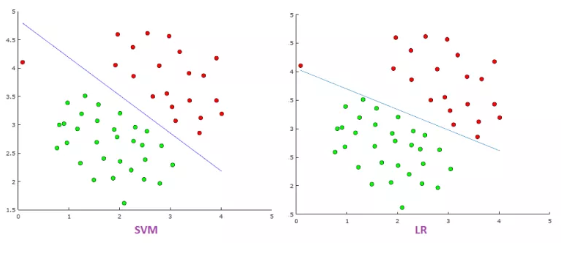LR’s boundary change by including one new example. In reality this may not be a good idea so being not sensitive like SVM tends to be better.
• Logistic Regression produces probabilistic values while SVM produces 1 or 0. So in a few words LR makes not absolute prediction and it does not assume data is enough to give a final decision. This maybe be good property when what we want is an estimation or we do not have high confidence into data.SVM hypothesis is a discriminator function producing 1 or 0Logistic Regression Hypothesis producing value close to 1 or 0 as probability like 0.23,0.76,0.89,0.99-Sigmoid functionHypothesis function

Usually, in order to get discrete values 1 or 0 for the LR we can say that when a function value is greater than a threshold we classify as 1 and when a function value is smaller than the threshold we classify as 0.

## General Advice

Try logistic regression first and see how you do with that simpler model. If logistic regression fails and you have reason to believe your data won’t be linearly separable, try an SVM with a non-linear kernel like a Radial Basis Function (RBF).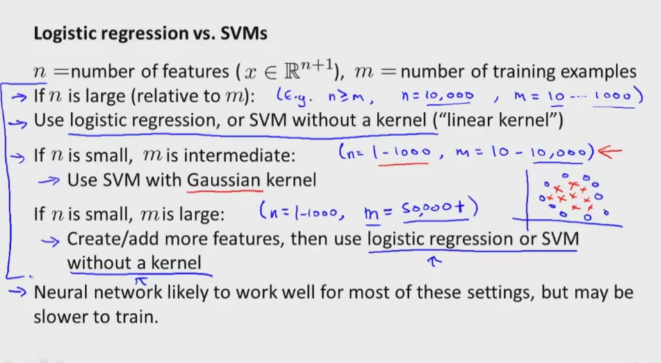Illustrates where to use each method SVM and Logistic Regression

## Take home message

• SVM tries to find the widest possible separating margin, while Logistic Regression optimizes the log likelihood function, with probabilities modeled by the sigmoid function.
• SVM extends by using kernel tricks, transforming datasets into rich features space, so that complex problems can be still dealt with in the same “linear” fashion in the lifted hyper space.

If you liked this article, please consider subscribing to my blog. That way I get to know that my work is valuable to you and also notify you for future articles.Thanks for reading and I am looking forward to hear your questions :)
Stay tuned and Happy Machine Learning.

Originally published at :https://towardsdatascience.com/support-vector-machine-vs-logistic-regression-94cc2975433f on Aug 12, 2018.